# Selina Solutions Concise Mathematics Class 6 Chapter 22: Simple (Linear) Equations Exercise 22(B)

Selina Solutions Concise Mathematics Class 6 Chapter 22 Simple (Linear) Equations Exercise 22(B) contains answers designed by expert faculty, in accordance with the exam pattern of the ICSE. The solutions are solved in a stepwise manner, as each step carries marks in the final examinations. Our set of faculty at BYJU’S prepare 100% accurate answers to boost the academic performance of students. Provided here, Selina Solutions Concise Mathematics Class 6 Chapter 22 Simple (Linear) Equations Exercise 22(B), free PDF links.

## Selina Solutions Concise Mathematics Class 6 Chapter 22: Simple (Linear) Equations Exercise 22(B) Download PDF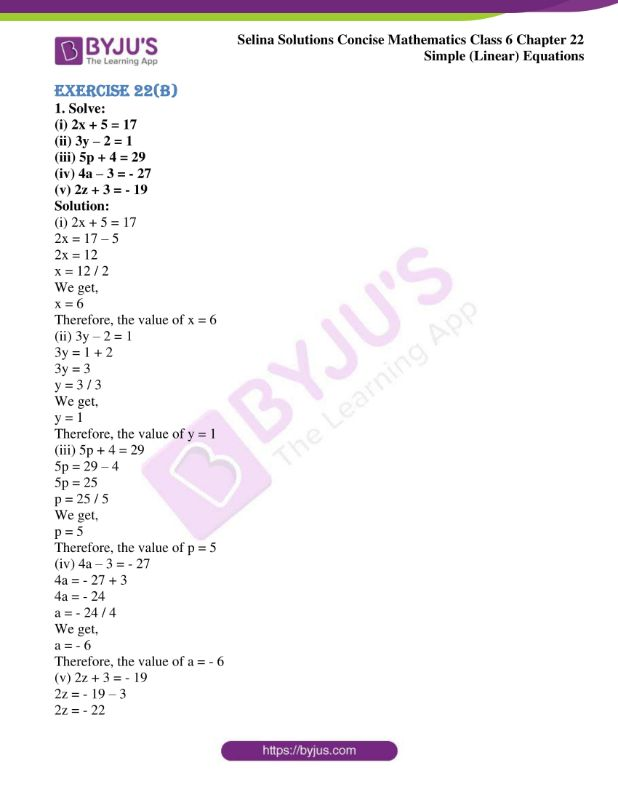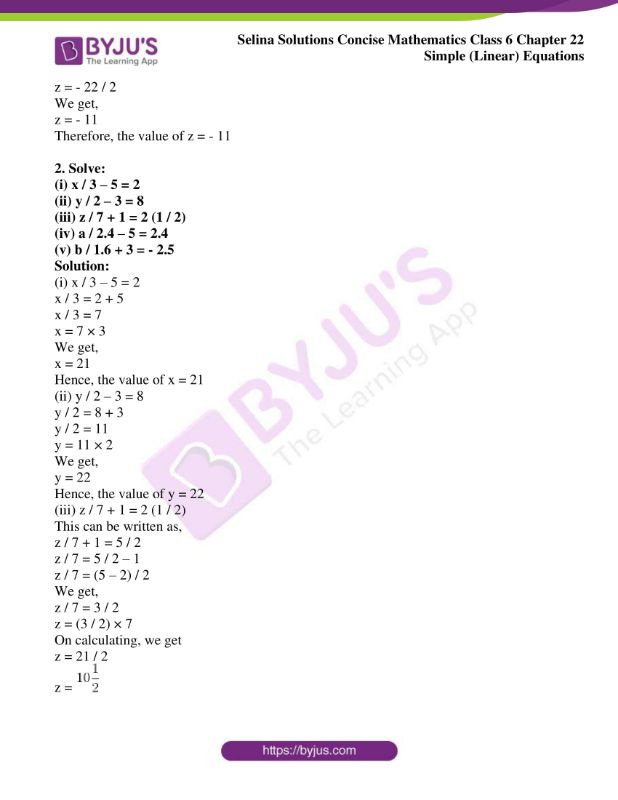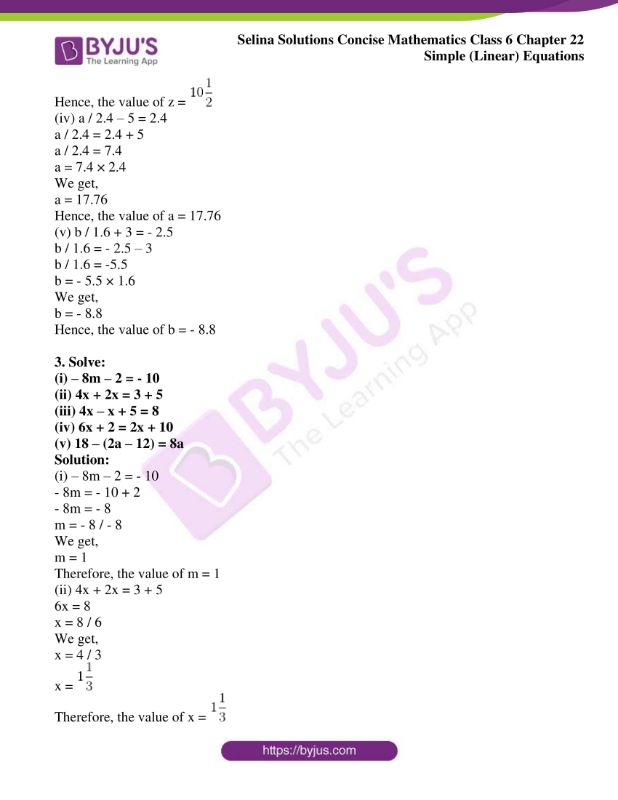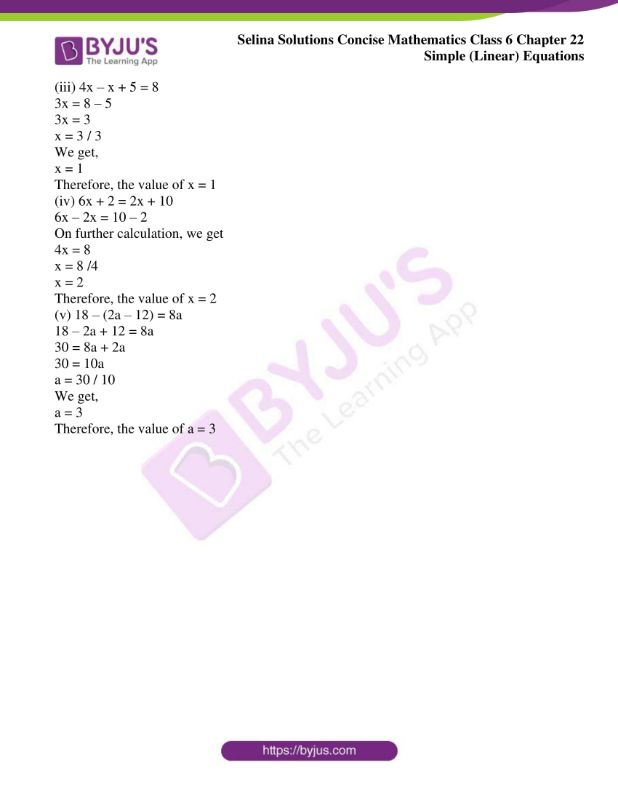### Access other exercises of Selina Solutions Concise Mathematics Class 6 Chapter 22: Simple (Linear) Equations

Exercise 22(A) Solutions

Exercise 22(C) Solutions

Exercise 22(D) Solutions

### Access Selina Solutions Concise Mathematics Class 6 Chapter 22: Simple (Linear) Equations Exercise 22(B)

Exercise 22(B)

1. Solve:

(i) 2x + 5 = 17

(ii) 3y – 2 = 1

(iii) 5p + 4 = 29

(iv) 4a – 3 = – 27

(v) 2z + 3 = – 19

Solution:

(i) 2x + 5 = 17

2x = 17 – 5

2x = 12

x = 12 / 2

We get,

x = 6

Therefore, the value of x = 6

(ii) 3y – 2 = 1

3y = 1 + 2

3y = 3

y = 3 / 3

We get,

y = 1

Therefore, the value of y = 1

(iii) 5p + 4 = 29

5p = 29 – 4

5p = 25

p = 25 / 5

We get,

p = 5

Therefore, the value of p = 5

(iv) 4a – 3 = – 27

4a = – 27 + 3

4a = – 24

a = – 24 / 4

We get,

a = – 6

Therefore, the value of a = – 6

(v) 2z + 3 = – 19

2z = – 19 – 3

2z = – 22

z = – 22 / 2

We get,

z = – 11

Therefore, the value of z = – 11

2. Solve:

(i) x / 3 – 5 = 2

(ii) y / 2 – 3 = 8

(iii) z / 7 + 1 = 2 (1 / 2)

(iv) a / 2.4 – 5 = 2.4

(v) b / 1.6 + 3 = – 2.5

Solution:

(i) x / 3 – 5 = 2

x / 3 = 2 + 5

x / 3 = 7

x = 7 × 3

We get,

x = 21

Hence, the value of x = 21

(ii) y / 2 – 3 = 8

y / 2 = 8 + 3

y / 2 = 11

y = 11 × 2

We get,

y = 22

Hence, the value of y = 22

(iii) z / 7 + 1 = 2 (1 / 2)

This can be written as,

z / 7 + 1 = 5 / 2

z / 7 = 5 / 2 – 1

z / 7 = (5 – 2) / 2

We get,

z / 7 = 3 / 2

z = (3 / 2) × 7

On calculating, we get

z = 21 / 2

z =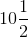Hence, the value of z =(iv) a / 2.4 – 5 = 2.4

a / 2.4 = 2.4 + 5

a / 2.4 = 7.4

a = 7.4 × 2.4

We get,

a = 17.76

Hence, the value of a = 17.76

(v) b / 1.6 + 3 = – 2.5

b / 1.6 = – 2.5 – 3

b / 1.6 = -5.5

b = – 5.5 × 1.6

We get,

b = – 8.8

Hence, the value of b = – 8.8

3. Solve:

(i) – 8m – 2 = – 10

(ii) 4x + 2x = 3 + 5

(iii) 4x – x + 5 = 8

(iv) 6x + 2 = 2x + 10

(v) 18 – (2a – 12) = 8a

Solution:

(i) – 8m – 2 = – 10

– 8m = – 10 + 2

– 8m = – 8

m = – 8 / – 8

We get,

m = 1

Therefore, the value of m = 1

(ii) 4x + 2x = 3 + 5

6x = 8

x = 8 / 6

We get,

x = 4 / 3

x =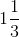Therefore, the value of x =(iii) 4x – x + 5 = 8

3x = 8 – 5

3x = 3

x = 3 / 3

We get,

x = 1

Therefore, the value of x = 1

(iv) 6x + 2 = 2x + 10

6x – 2x = 10 – 2

On further calculation, we get

4x = 8

x = 8 /4

x = 2

Therefore, the value of x = 2

(v) 18 – (2a – 12) = 8a

18 – 2a + 12 = 8a

30 = 8a + 2a

30 = 10a

a = 30 / 10

We get,

a = 3

Therefore, the value of a = 3